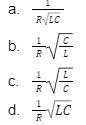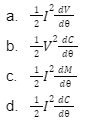# Electrical Engineering MCQs

Solve Multiple-Choice Questions on Electrical Engineering to prepare better for the upcoming competitive exams. It is very beneficial to solve MCQs before the exams for multiple reasons. You can practise, analyse and understand concepts while solving them. It will also help you strengthen your time management skills.

Electrical Engineering MCQs are a prerequisite to the current examination pattern. If you wish to learn more about MCQs, you can check notes, mock tests, and previous years’ question papers. Get an idea about Electrical Engineering MCQs by solving the ones compiled below for your practice.

### Electrical Engineering Multiple-Choice Questions

1. Magnesium vapour in a filament lamp gives
1. Green colour light
2. Yellow colour light
3. Blue colour light
4. Red colour light

2. In a series resonance circuit at resonance circuit at resonance, selectivity is equal to
3.4. The average value of the voltage wave V = 110 + sin (314t – 25o) volts is
1. 110 V
2. 200 V
3. 177 V
4. 220 V

5. The materials to be used in manufacture of a standard resistance should be
1. High resistivity and low temperature coefficient
2. Low resistivity
3. High temperature
4. None of the above

6. A 2 kVA transformer has iron loss of 150 W and full-loss copper loss of 250 W. The maximum efficiency of the transformer would occur when the total loss is
1. 450 W
2. 400 W
3. 250 W
4. 300W

7. Why damping is provided in an instrument for
1. Increasing the range
2. Reducing the settling time
3. Both (a) and (b)
4. Neither (a) nor (b)

8. What does the size of the transformer core depend on?
1. Frequency
2. Permissible flux density in the core material
3. Area of the core
4. Both (a) and (b)

9. If the applied voltage to a dc motor is 230 V then the back emf, for maximum power developed is
1. 115 V
2. 220 V
3. 300 V
4. 500 V

10. The full-load speed of a 6-pole, 50 Hz induction motor is 950 rpm, what is its half-load speed nearly equal to?
1. 990 rpm
2. 1800 rpm
3. 475 rpm
4. 975 rpm

11. For 66 kV lines the no. of insulator disc are used
1. 2
2. 6
3. 8
4. 10

12. The p.u. synchronous impedance of a machine is 20 p.u., its Short Circuit Ratio (SCR) is
1. 3
2. 1.441
3. 0.25
4. 0.5

13. The zero sequence reactance will be more than the positive sequence reactance, if the equipment is a
1. Generator
2. Transformer
3. Neither (a) nor (b)
4. Both (a) and (b)

14. For highly dropped diode
1. Zener breakdown is likely to take place
2. Avalanche breakdown is likely to take place
3. Both (a) and (b)
4. Neither (a) nor (b)

15. Two Conductors A and B of the same material and length l and 2l have radius r and 2r, respectively. The ratio of their specific resistance will be
1. 1:3
2. 1:1
3. 1:4
4. 1:5

16. The average value of a half wave rectified voltage with a peak value of 200 V is
1. 250 V
2. 125 V
3. 144.5 V
4. 63.7 V

17. Which of the following theorems is applicable for both linear and non linear circuits?
1. Superposition
2. Thevenin’s
3. Norton’s
4. None of the above

18. For (Overhead) OH transmission line, the self GMD method is used to find
1. Capacitance
2. Induction
3. Both (a) and (b)
4. Neither (a) nor (b)

19. If the length of a bar of magnetic material is increased by 20% and the cross-sectional area is decreased by 20% the reluctance is
1. Decreased by 33%
2. Increased by 55%
3. Decreased by 55%
4. Increased by 50%

20. The maximum power that a 12 V dc source with an internal resistance of 2 ohm can supply to resistive load is
1. 15 W
2. 18 W
3. 35 W
4. 45 W

21. Which one of the following statements is correct? The defleq hot-wire instrument depends on
1. RMS value of the AC current
2. RMS value of the AC voltage
3. Average value of the AC current
4. Average value of the AC voltage

22. Which one of the following meters is an integrating type of instrument?
1. Ammeter
2. Voltmeter
3. Wattmeter
4. Energy meter

23. The developed torque of an electrostatic voltmeter is given by
24.25. If the applied voltage of a certain transformer is increased by 50% and the frequency is reduced to 50% (assuming that the magnetic circuit remains unsaturated), the maximum core flux density will
1. Change to 3 times the original value
2. Change to 2 times the original value
3. Change to 1.5 times the original value
4. Remains same as the original value

26. For successful parallel operation of two single phase transformers, the most essential condition is that their
1. Percentage impedance are equal
2. Polarities are properly connected
3. Turns-ratio are exactly equal
4. kVA ratings are equal